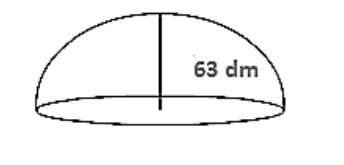# The dome of a building is in the form of a hemisphere. Its radius is 63dm.

Question:

The dome of a building is in the form of a hemisphere. Its radius is 63dm. Find the cost of painting it at the rate of Rs.2 per sq m.

Solution:

Dome radius = 63 dm = 6.3 m

Inner surface area of dome $=2 \pi r^{2}$

$=2 \times 3.14 \times(6.3)^{2}$

$=249.48 \mathrm{~m}^{2}$

Now, cost of $1 \mathrm{~m}^{2}=$ Rs. 2

Therefore cost of $249.48 \mathrm{~m}^{2}=$ Rs. $(249.48 \times 2)=$ Rs. $498.96$